# How To Tackle Oblique Projection Assignment Questions With Ease

Apart from helping you complete your assignment on time, we strive to equip you with the knowledge you need to tackle oblique projection assignments easily. We have posted below oblique projections assignment models along with stellar-quality solutions to aid your revision. The sample questions and answers will give you an idea of how to tackle oblique projection assignments. Remember, our seasoned architecture assignment tutors are at your service 24x7. You can hire them at your convenience. Your quest for a reliable oblique projection assignment help service should end here with us.

## What is an oblique projection?

A pictorial view of an object can be produced in oblique projection. where the front face is sketched as a true shape without distortion. Sketching in this projection is much easier than in isometric projection since all the circles in the front face are sketched as plane figures of ellipses as in isometric projection.
Oblique sketching starts with two axes one vertical and one horizontal together with a third axis which is usually drawn at 45" to the horizontal and along which all measurements are reduced to half true length (TL), as shown in Fig. 4.23. This projection is sometimes called a 'cabinet' projection.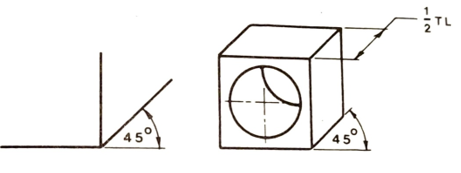Fig. 4.23 Basic oblique axes and 'cabinet' projection

All proportions and measurements can be made only along these three axes.

A comparison of three pictorial projections is shown in Fig. 4.24.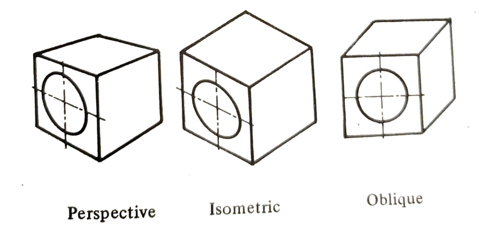Fig. 4.24 Pictorial projections

## How do you draw an oblique projection?

The main advantage of oblique projection over isometric is that any complicated face - which may be curved, irregular, or has several holes can be drawn as its true shape using drawing instruments.

The receding surfaces can be drawn at any angle but are usually drawn at 45 and are foreshortened to half-true length.

All receding surfaces shown in Fig. 4.25 are half true length but at different angles.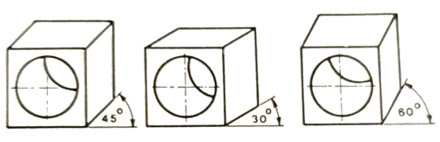Fig. 4.25 Receding surfaces at different angles

In Fig. 4.26 the required semicircles are drawn with centers at A. B and C are positioned along a line at 45" and distances of half-true lengths.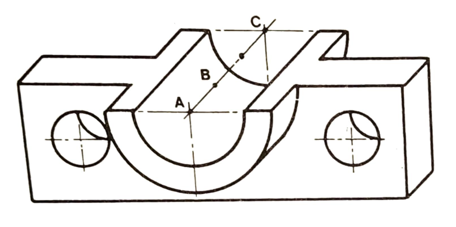Fig. 4.26 Bracket in oblique projection

Figure 4.27 indicates the advantages of oblique projection when drawing a complicated cylindrical component.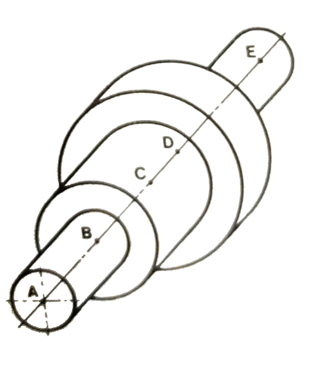Fig. 4.27 Cylindrical component in oblique projection

## What are the steps of drawing cylindrical shapes in oblique projection?

1. Draw the axis of the cylindrical shape at 45" to the horizontal and locate the points A, B, C, and D representing all normal (at 90") surfaces along the axis at distances of half true length.
2.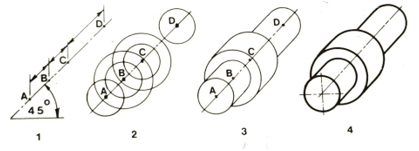Fig. 4.28 Drawing cylindrical shapes

3. Draw the required construction circles at the points A, B, C, and D.
4. Join adjacent pairs of same-size circles by tangents at 45°.
5. To complete, draw all circles and blend tangents with uniform bold lines as shown.

Figure 4.29 shows how complicated cylindrical shapes can be represented in oblique projection.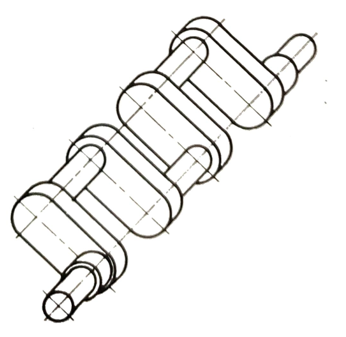Fig. 4.29 Crankshaft in oblique projection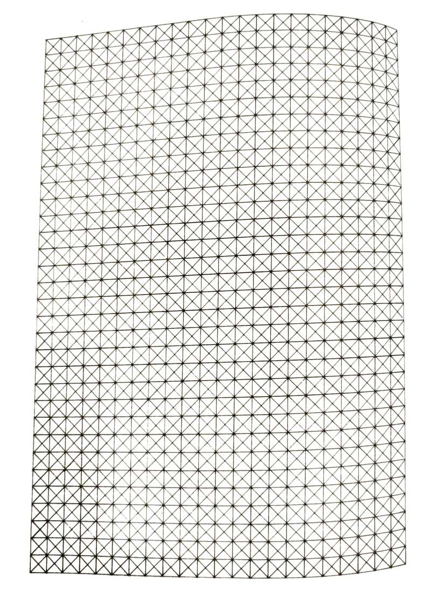Fig. 4.30 Oblique grid for guided sketching